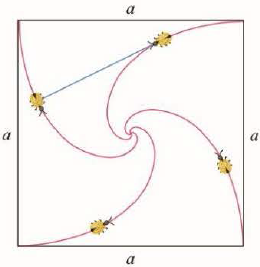Chapter 10, Problem 4P

Chapter
Section
Textbook Problem

# Four bugs are placed at the four comers of a square with side length a. The bugs crawl counterclockwise at the same speed and each bug crawls directly toward the next bug at all times. They approach the center of the square along spiral paths. (a) Find the polar equation of a bug’s path assuming the pole is at the center of the square. (Use the fact that the line joining one bug to the next is tangent to the bug’s path.) (b) Find the distance traveled by a bug by the time it meets the other bugs at the center. FIGURE FOR PROBLEM 4(a)

To determine

To find: The polar equation of the bug’s path.

Explanation

Calculation:

Assume the bug starts in the upper right corner of the square, so the polar coordinates are (r,θ) and it crawling towards (r,θ+π2) .

Calculate the value of x.

x=rcosθ

Substitute θ+π2 for θ in the above equation.

x=rcosθx=rcos(θ+π2)x=rsinθ

Calculate the value of y.

y=rsinθ

Substitute θ+π2 for θ in the above equation.

y=rsinθy=rsin(θ+π2)y=rcosθ

Use the equation,

(dr/dθ)sinθ+rcosθ(dr/dθ)cosθrsinθ=sinθcosθsinθ+cosθ(drdθ)sinθ+rcosθ(sinθ+cosθ)=(drdθ)cosθrsinθ(sinθcosθ)(drdθ)sin2θ+(drdθ)sinθcosθ+rcosθsinθ+rcos2θ=[(drdθ)cosθsinθ(drdθ)cos2θrsin2θ+rsinθcosθ][(drdθ)</

(b)

To determine

To find: The distance travelled by a bug.

### Still sussing out bartleby?

Check out a sample textbook solution.

See a sample solution

#### The Solution to Your Study Problems

Bartleby provides explanations to thousands of textbook problems written by our experts, many with advanced degrees!

Get Started

#### Find the domain of the function. a. f(x) = 9x b. f(x) = x+32x2x3

Applied Calculus for the Managerial, Life, and Social Sciences: A Brief Approach

#### Find the derivative of the function. y=x+x+x

Single Variable Calculus: Early Transcendentals

#### True or False: A trigonometric substitution is necessary to evaluate xx2+10dx.

Study Guide for Stewart's Single Variable Calculus: Early Transcendentals, 8th